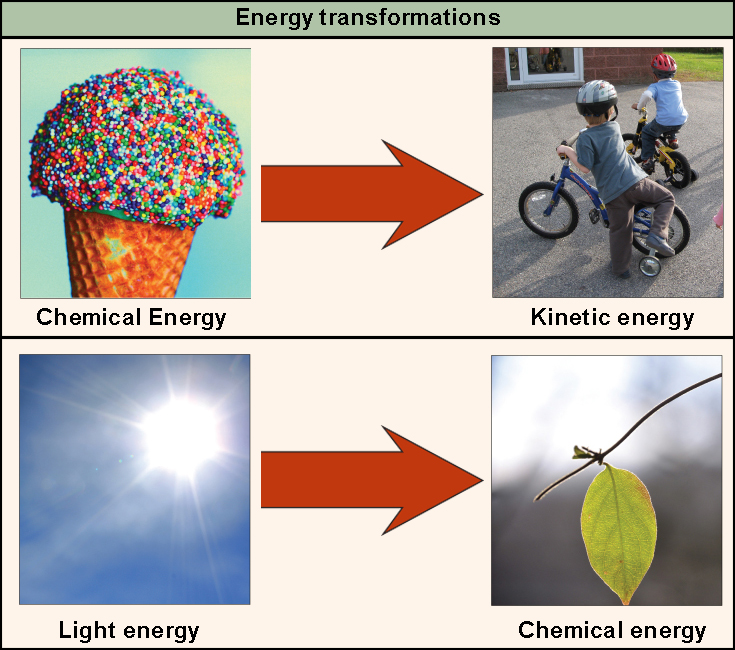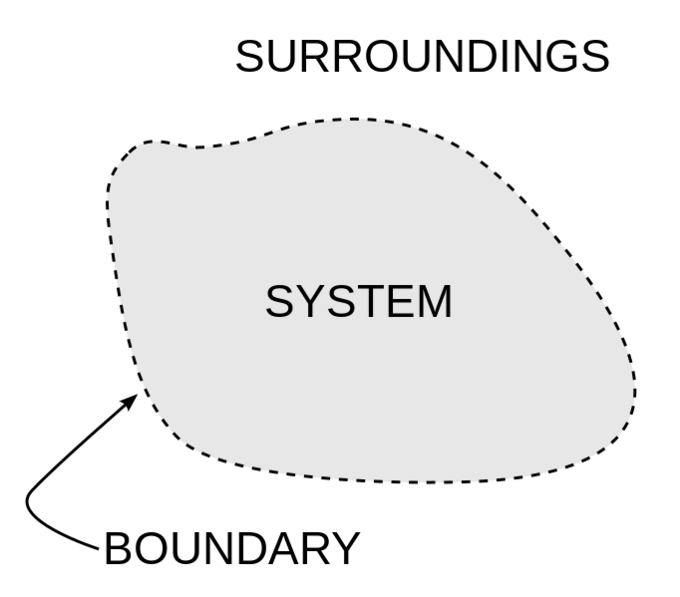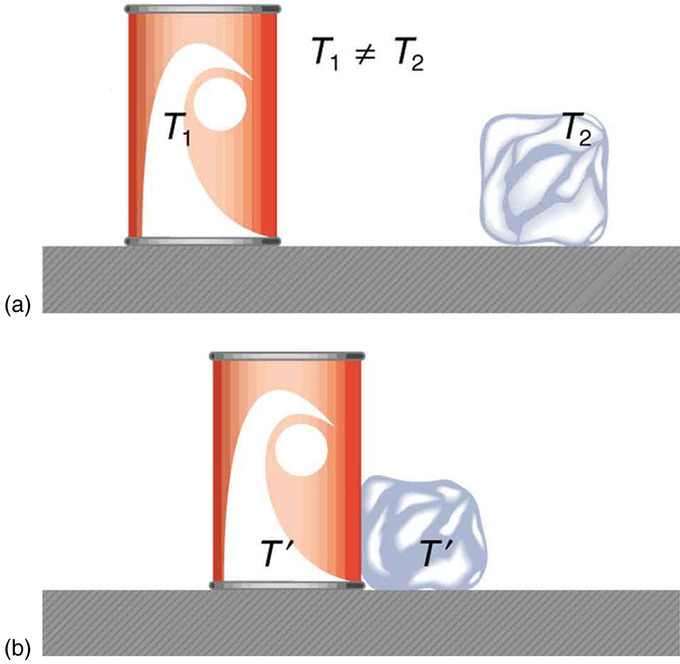## The First Law of Thermodynamics

The first law of thermodynamics states that energy can be transferred or transformed, but cannot be created or destroyed.

### Learning Objectives

Describe the first law of thermodynamics

### Key Takeaways

#### Key Points

• According to the first law of thermodynamics, the total amount of energy in the universe is constant.
• Energy can be transferred from place to place or transformed into different forms, but it cannot be created or destroyed.
• Living organisms have evolved to obtain energy from their surroundings in forms that they can transfer or transform into usable energy to do work.

#### Key Terms

• first law of thermodynamics: A version of the law of conservation of energy, specialized for thermodynamical systems, that states that the energy of an isolated system is constant and can neither be created nor destroyed.
• work: A measure of energy expended by moving an object, usually considered to be force times distance. No work is done if the object does not move.

Thermodynamics is the study of heat energy and other types of energy, such as work, and the various ways energy is transferred within chemical systems. “Thermo-” refers to heat, while “dynamics” refers to motion.

### The First Law of Thermodynamics

The first law of thermodynamics deals with the total amount of energy in the universe. The law states that this total amount of energy is constant. In other words, there has always been, and always will be, exactly the same amount of energy in the universe.

Energy exists in many different forms. According to the first law of thermodynamics, energy can be transferred from place to place or changed between different forms, but it cannot be created or destroyed. The transfers and transformations of energy take place around us all the time. For instance, light bulbs transform electrical energy into light energy, and gas stoves transform chemical energy from natural gas into heat energy. Plants perform one of the most biologically useful transformations of energy on Earth: they convert the energy of sunlight into the chemical energy stored within organic molecules.The first law of thermodynamics: Shown are two examples of energy being transferred from one system to another and transformed from one form to another. Humans can convert the chemical energy in food, like this ice cream cone, into kinetic energy by riding a bicycle. Plants can convert electromagnetic radiation (light energy) from the sun into chemical energy.

### The System and Surroundings

Thermodynamics often divides the universe into two categories: the system and its surroundings. In chemistry, the system almost always refers to a given chemical reaction and the container in which it takes place. The first law of thermodynamics tells us that energy can neither be created nor destroyed, so we know that the energy that is absorbed in an endothermic chemical reaction must have been lost from the surroundings. Conversely, in an exothermic reaction, the heat that is released in the reaction is given off and absorbed by the surroundings. Stated mathematically, we have:

$\Delta E=\Delta E_{sys}+\Delta E_{surr}=0$The system and surroundings: A basic diagram showing the fundamental distinction between the system and its surroundings in thermodynamics.

### Heat and Work

We know that chemical systems can either absorb heat from their surroundings, if the reaction is endothermic, or release heat to their surroundings, if the reaction is exothermic. However, chemical reactions are often used to do work instead of just exchanging heat. For instance, when rocket fuel burns and causes a space shuttle to lift off from the ground, the chemical reaction, by propelling the rocket, is doing work by applying a force over a distance.

If you’ve ever witnessed a video of a space shuttle lifting off, the chemical reaction that occurs also releases tremendous amounts of heat and light. Another useful form of the first law of thermodynamics relates heat and work for the change in energy of the internal system:

$\Delta E_{sys}=Q+W$

While this formulation is more commonly used in physics, it is still important to know for chemistry.Rocket launch: The powerful chemical reaction propelling the rocket lets off tremendous heat to the surroundings and does work on the surroundings (the rocket) as well.

## Heat and Work

Both heat and work refer to processes by which energy is transferred to or from a substance.

### Learning Objectives

Define heat and work

### Key Takeaways

#### Key Points

• When energy is exchanged between thermodynamic systems by thermal interaction, the transfer of energy is called heat.
• Work is the transfer of energy by any process other than heat.
• Heat and work are related: work can be completely converted into heat, but the reverse is not true: heat cannot be completely converted to work.

#### Key Terms

• heat: The energy transferred from one system to another by thermal interaction.
• work: The transfer of energy by any process other than heat.
• thermochemistry: The study of the energy and heat associated with chemical reactions and/or physical transformations.

### Definition of Heat

When energy is exchanged between thermodynamic systems by thermal interaction, the transfer of energy is called heat. The units of heat are therefore the units of energy, or joules (J). Heat is transferred by conduction, convection, and/or radiation.

Heat is transfer by conduction occurs when an object with high thermal energy comes into contact with an object with low thermal energy. Heat transfer by convection occurs through a medium. For example, when heat transfers from the hot water at the bottom of the pot to the cooler water at the top of the pot. Lastly, heat can also be transferred by radiation; a hot object can convey heat to anything in its surroundings via electromagnetic radiation.

When a high temperature body is brought into contact with a low temperature body, the temperatures equilibrate: there is heat flow from higher to lower temperature, like water flowing downhill, until the temperatures of the bodies are equivalent. The high temperature body loses thermal energy, and the low temperature body acquires this same amount of thermal energy. The system is then said to be at thermal equilibrium.An illustration of thermal equilibrium: The can of cola and ice cube start at different temperatures. When they come into contact, heat is transferred from the cola can to the ice cube until both bodies reach thermal equilibrium.

### Definition of Work

Work is the transfer of energy by any process other than heat. Like heat, the unit measurement for work is joules (J). There are many forms of work, including but not limited to mechanical, electrical, and gravitational work. For our purposes, we are concerned with P-V work, which is the work done in an enclosed chemical system. In this type of system, work is defined as the change in the volume (V) in liters within the system multiplied by a pressure (P). Assuming the system is at constant pressure, this equates to the following:

$W=P\Delta V$

Most often, we are interested in the work done by expanding gases. Assuming the gases are ideal, we can apply the ideal gas law to the above equation to get the following:

$W=P\Delta V=nR\Delta T$

### Relationship Between Heat and Work

Heat and work are related. Work can be completely converted into heat, but the reverse is not true: heat energy cannot be wholly transformed into work energy. Scientists and engineers have been able to exploit the principles of thermochemistry to develop technologies ranging from hot/cold packs to gasoline powered combustion engines.

For a closed system, the change in internal energy (∆U) is related to heat (Q) and work (W) as follows:

$\Delta U = Q+W$

This means that the total energy within a system is affected by the sum of two possible energy transfers: heat and work.